﻿ 清江流域降水的多模式BMA概率预报试验
 快速检索 中文标题 英文标题 作者中文名 作者英文名 单位中文名 单位英文名 中文关键词 英文关键词 中文摘要 英文摘要 基金项目 全文
 气象2020, Vol. 46Issue (1): 108-118.  DOI: 10.7519/j.issn.1000-0526.2020.01.011### 引用本文 [复制中英文]

[复制中文]
QI Haixia, PENG Tao, LIN Chunze, et al, 2020. Probabilistic Forecasting of Precipitation over the Qingjiang River Basin Using BMA Multimodel Ensemble Technique[J]. Meteorological Monthly, 46(1): 108-118. DOI: 10.7519/j.issn.1000-0526.2020.01.011.
[复制英文]

### 文章历史

2018年8月22日收稿
2019年11月5日收修定稿

1. 中国气象局武汉暴雨研究所暴雨监测预警湖北省重点实验室，武汉 430205
2. 南京信息工程大学气象灾害教育部重点实验室/气象灾害预报预警与评估协同创新中心，南京 210044
3. 武汉区域气候中心，武汉 430074
4. 武汉农业气象试验站，武汉 430040

Probabilistic Forecasting of Precipitation over the Qingjiang River Basin Using BMA Multimodel Ensemble Technique
, PENG Tao1, LIN Chunze1, PENG Ting2, JI Luying2, LI Lan3, MENG Cuili4
1. Hubei Key Laboratory for Heavy Rain Monitoring and Warning Research, Institute of Heavy Rain, CMA, Wuhan 430205;
2. Key Laboratory of Meteorological Disaster, Ministry of Education (KLME)/Collaborative Innovation Center on Forecast and Evaluation of Meteorological Disasters (CIC-FEMD), Nanjing University of Information Science and Technology, Nanjing 210044;
3. Wuhan Regional Climate Center, Wuhan 430074;
4. Wuhan National Agrometeorological Station, Wuhan 430040
Abstract: Based on the TIGGE datasets from the European Centre for Medium Range Weather Forecasts (ECMWF), the United Kingdom Met Office (UKMO), the China Meteorological Administration (CMA), and the Japan Meteorological Agency (JMA), and its multi-center ensemble systems, and observations in the Qingjiang River Basin, Bayesian model averaging (BMA) probability forecast models were established. The results showed that the optimal length of the training period is about 40 days, and the BMA models for multi-center ensemble outperform those for single center system for lead times of 24 h. The mean absolute error (MAE) and continuous ranked probability score (CRPS) skills of the BMA models are improved approximately 11% and 15%, respectively, compared with those of raw ensemble forecasts. In operation, when the BMA 90 percentile predicted precipitation is extreme precipitation [[50-100) mm·(24 h)-1], the 75-90 percentiles predicted precipitation could be used as the forecast reference, and the heavy precipitation warning could be carried out. For the forecast of severe precipitation [[50-100) mm·(24 h)-1], the forecast result of the 50-75 percentile predicted by BMA can be taken as a reference, while for the general precipitation [≤25 mm·(24 h)-1], the reference of BMA deterministic forecast is relatively strong. BMA probability forecast could give both the PDF curve with full probability and the probability greater than a certain precipitation intensity, which could provide the basis for the probability forecast in operation. However, the small probability value is often ignored, resulting in omission. So how to capture more useful information through the probabilistic prediction method and increase the accuracy of the prediction of extreme weather events will be a challenge for the probabilistic prediction technology.
Key words: TIGGE    Bayesian model averaging (BMA)    multimodel ensemble    probalility forecast

1 贝叶斯模型平均(BMA) 1.1 多模式集合降水预报的BMA模型

 $p\left(y | f_{1}, \cdots, f_{K}\right)=\sum\limits_{k=1}^{K} w_{k} g_{k}\left[y |\left(f_{k}, y^{T}\right)\right]$ (1)

 \begin{aligned} \operatorname{logit}[P(y&\left.\left.=0 | f_{k}\right)\right] \equiv \log \frac{P\left(y=0 | f_{k}\right)}{P\left(y>0 | f_{k}\right)} \\ &=a_{0 k}+a_{1 k} f_{k}^{\frac{1}{3}}+a_{2 k} \delta_{k} \end{aligned} (2)

 $g_{k}\left(y | f_{k}\right)=\frac{1}{\beta_{k}^{\alpha} \varGamma\left(\alpha_{k}\right)} y^{\alpha_{k}-1} \exp \left(-y / \beta_{k}\right)$ (3)
 \begin{aligned} &\mu_{k}=\alpha_{k} \beta_{k}=b_{0 k}+b_{1 k} f_{k}^{1 / 3}\\ &\sigma_{k}^{2}=\alpha_{k} \beta_{k}^{2}=c_{0}+c_{1} f_{k} \end{aligned} (4)

 $l\left(w_{1}, \cdots, w_{k} ; c_{0}, c_{1}\right)=\sum\limits_{t} \log p\left(y_{s t} | f_{1 s t}, \cdots f_{K s t}\right)$ (5)

EM算法是用于存在未知变量的情况下查找最大似然因子的算法。为便于计算，引入未知变量zkst，其取值规则：当第k个成员是最佳预报结果时，其值为1；若第k个成员的预报结果不是最佳预报结果时，其值为0。在实际计算过程中zkst的值并不一定是整数。EM算法是一个两步迭代算法。待估参数会首先给定一个合理的初值估计，然后再进行迭代。迭代分为两步进行：

 $\begin{array}{c} h_{k}\left(y | f_{k}\right)= P\left(y=0 | f_{k}\right) I(y=0)+\\ P\left(y>0 | f_{k}\right) g_{k}\left(y | f_{k}\right) I(y>0) \end{array}$ (6)

 $p\left(y | f_{1}, \cdots, f_{K}\right) =\sum\limits_{k=1}^{K} w_{k}\left[P\left(y=0 | f_{k}\right) \times\right.\\ I(y=0)+P\left(y>0 | f_{k}\right) g_{k}\left(y | f_{k}\right) \times \\ I(y>0)]$ (7)

1.2 BMA概率预报检验与评估方法

 $M A E=\frac{1}{N} \sum\limits_{i=1}^{N}\left|f_{i}-o_{i}\right|$ (8)

CRPS评分可看作是阈值连续化之后BS评分(Brier，1950)在所有可能阈值上的积分。设Fi(x)为预报累积概率分布函数(CDF)，x是降水预报值，oi是降水观测值，CRPS可表示为：

 $C R P S=\frac{1}{N} \sum\limits_{i=1}^{N} \int_{-\infty}^{+\infty}\left[F_{i}(x)-H\left(x-o_{i}\right)\right] \mathrm{d} x$ (9)

2 研究区域与资料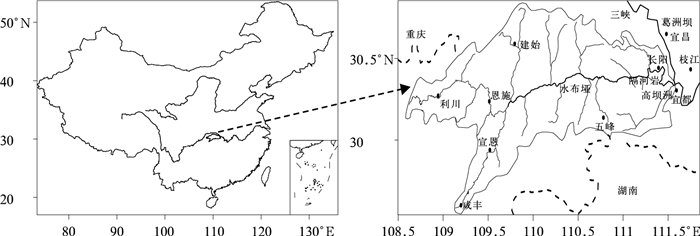图 1 清江流域及10个国家基准气象观测站(黑点)分布 Fig. 1 Distribution of the Qingjiang River Basin and the location (dots) of 10 standard meteorological stations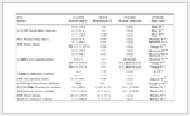表 1 TIGGE四个全球集合预报系统(ECMWF，NCEP，UKMO，CMA)基本信息 Table 1 Description of four ensemble prediction systems in TIGGE in this study (ECMWF, UKMO, CMA, JMA)
3 结果

3.1 训练期长度选取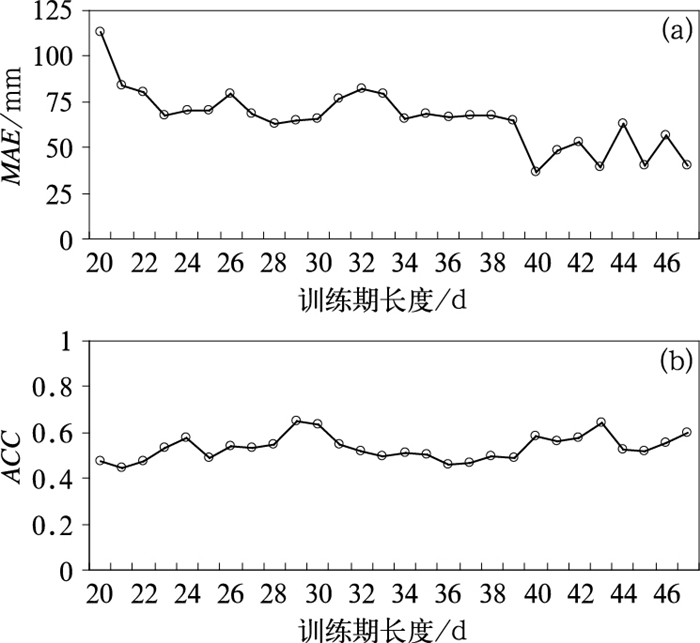图 2 多模式集合的BMA模型预报的MAE(a)、ACC(b)随训练期长度的变化 Fig. 2 Comparison of BMA predictive models of average daily precipitation (a) MAE, (b) ACC for different training period lengths
3.2 各集合模式权重对比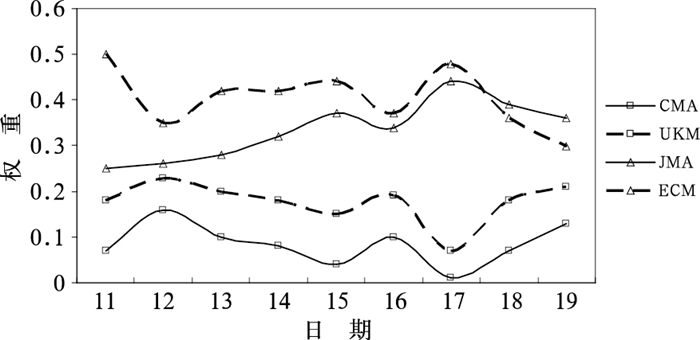图 3 2016年7月11—19日预报期内各集合模式的权重对比 Fig. 3 BMA weights of the four models over the prediction period for precipitation during 11-19 July 2016
3.3 BMA概率预报检验与评估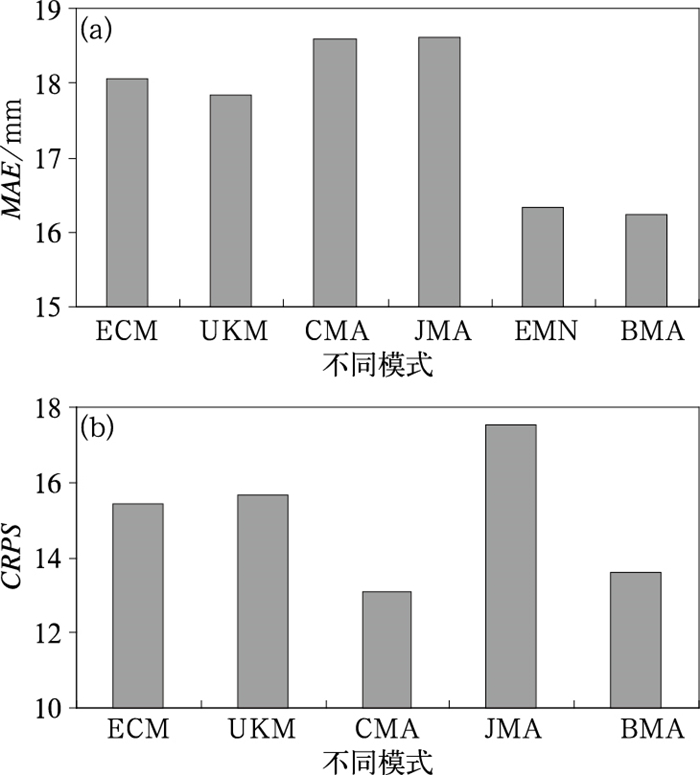图 4 所有站点的多模式集合BMA预报及原始单模式集合预报的MAE(a)、CRPS(b)均值对比 Fig. 4 Comparison of MAE means (a) and CRPS means (b) of BMA predictive models and four models ensemble forecasts of daily precipitation
3.4 BMA概率预报分析表 2 2016年7月18日建始站以及7月17日利川站BMA模型预报的效果评估 Table 2 BMA probabilistic quantitative forecasts, four models ensemble forecasts, logistic regression PoP, percentile forecasts and observations at Jianshi Station on 18 and Lichuan Station on 17 July 2016

BMA概率预报可以产生高度集中的概率密度函数PDF曲线。PDF曲线越尖，区间范围越窄，说明BMA预报可以将实际大气可能发生状态缩小在一个更小的区间范围内，预报的不确定性也更小。图 5给出7月18日建始站及17日利川站24 h预报时效BMA预报降水的PDF。BMA预报的PDF(黑色曲线)是四个集合模式PDF(蓝色曲线)的加权结果。由图 5a看出，对于极端降水事件(大暴雨量级以上)，实况降水(黑色垂直线)远远超出了四个集合模式(黑色空心圈)的预报结果，但是BMA预报的PDF曲线却能很好地将其包含在内，没出现漏报现象，但是结果接近90百分位预报。而对于一般性降水(大雨量级以下，图 5b)，经过BMA集合后的PDF曲线较三个集合模式曲线更尖更窄，缩小了预报结果的不确定性。其中一个模式预报的PDF曲线区间范围较BMA预报要小，但是从确定性预报结果看，BMA确定性预报与实况最为接近。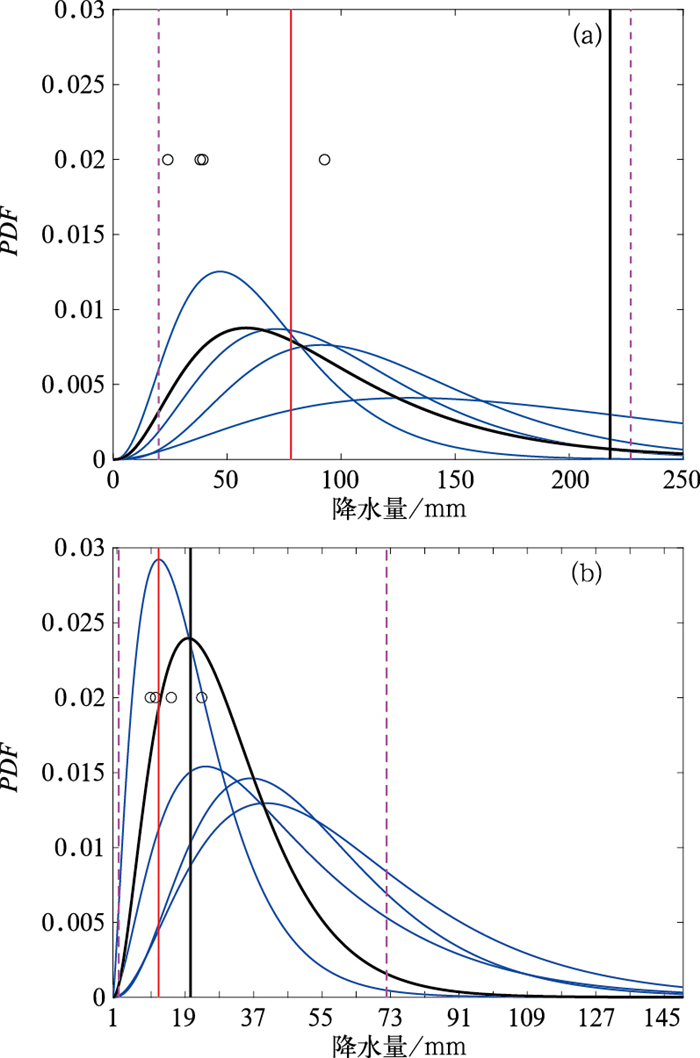图 5 2016年7月18日建始站(a)和17日利川站(b)24 h预报时效24 h累积降水BMA预报PDF曲线 (黑色曲线：BMA预报PDF，蓝色曲线：四个集合模式预报PDF，黑色垂直实线：实况，黑色空心圈：四个集合成员预报，红色垂直实线：BMA的50百分位预报，粉色垂直虚线：BMA的10和90百分位预报) Fig. 5 BMA predictive PDF of daily precipitation at (a) Jianshi Station on 18 and (b) Lichuan Station on 17 July 2016 (black solid curve: BMA predictive PDF; blue solid curve: four models' predictive PDF; black thick vertical line: observation; red vertical line: deterministic forecast; dashed pink vertical lines: the 10th percentile and 90th percentile forecasts from the BMA predictive PDF)

3.5 百分位预报法及极端降水分析

BMA概率预报给出的是全概率PDF结果，其包含了集合预报不确定性的定量估计。刘建国等(2013)通过BMA概率预报方法分析了高温极端天气事件信息，并提出基于集合预报的概率分布来预警高温天气的方案。本文根据BMA预报PDF给出百分位预报结果，研究分析多模式集合的BMA百分位预报法在极端事件或一般性事件中的适用性，探讨如何捕捉PDF中有用信息，并探讨其局限性。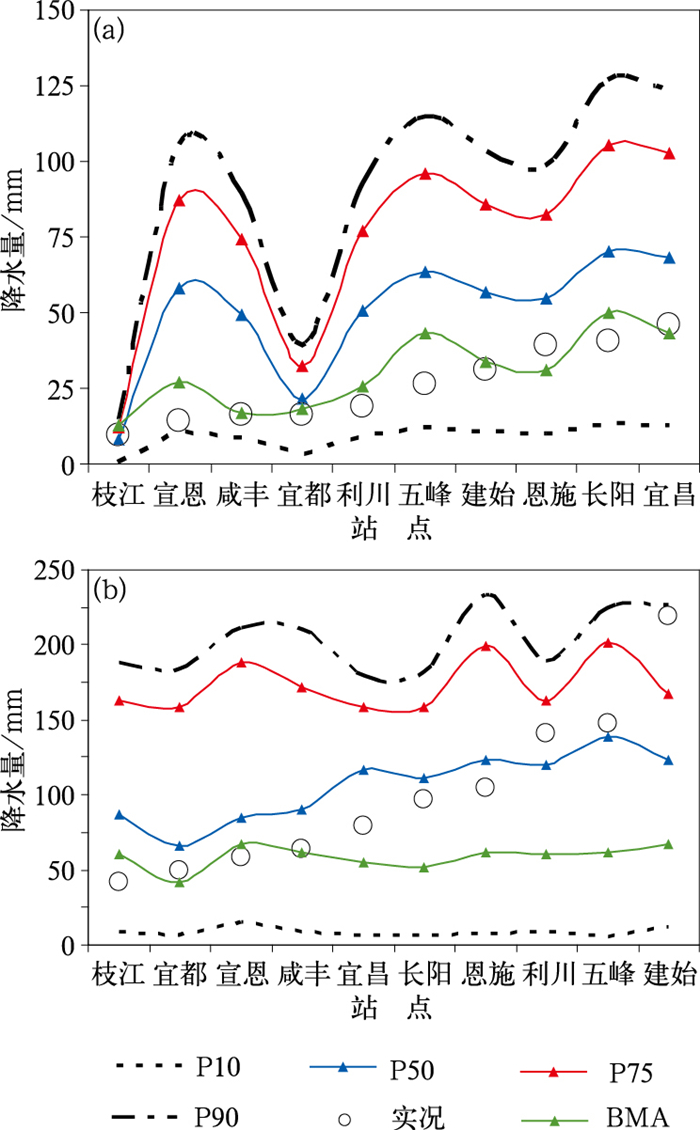图 6 2016年7月17日(a)、18日(b)清江流域10个站24 h预报时效降水的BMA百分位预报和观测 (P10、P50、P75、P90曲线分别为经过PDF计算得到的10、50、75以及90百分位数预报值，BMA曲线为确定性预报结果) Fig. 6 Percentile forecasts (P10, P50, P75, P90) and observations of average precipitation at all stations in Qingjiang River Basin on 17 (a) and 18 (b) July 2016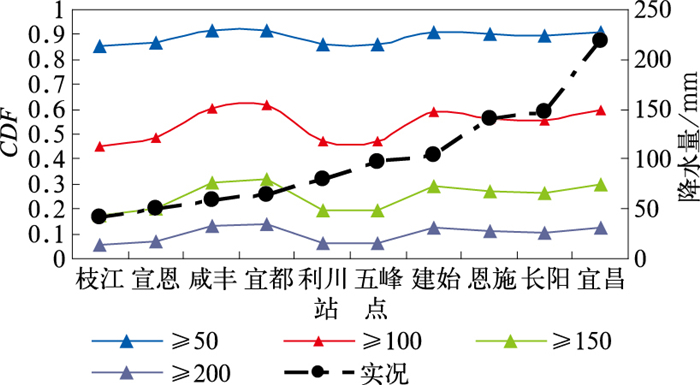图 7 2016年7月18日清江流域10个站24 h预报时效日降水BMA预报超过50、100、150以及200 mm的概率与观测 Fig. 7 Probability of exceeding 50 mm and observations for 24 h precipitation at all 10 stations Qingjiang River Basin on 18 July 2016
4 结论与讨论

(1) 在清江流域开展TIGGE多模式集合的BMA概率降水预报试验，通过多组训练期长度敏感性试验分析表明，训练期长度选择40 d较为合适。

(2) 多模式集合BMA预报比原始集合预报有更高预报技巧，比四个原始集合预报MAE平均值减少近11%左右，而对于CRPS除了CMA模式无订正效果外，较其他三个模式平均值提高近15%左右。

(3) BMA预报既能给出确定性预报结果也可以给出概率预报结果。对于极端强降水，BMA的75~90百分位数预报效果较好，对于强降水，BMA的50~75百分位数预报效果较好，对于一般性降水，BMA确定性预报结果或50百分位数预报效果较好。

 陈朝平, 冯汉中, 陈静, 2010. 基于贝叶斯方法的四川暴雨集合概率预报产品释用[J]. 气象, 36(5): 32-39. Chen C P, Feng H Z, Chen J, 2010. Application of Sichuan heavy rainfall ensemble prediction probability products based on Bayesian method[J]. Meteor Mon, 36(5): 32-39 (in Chinese). DOI:10.3969/j.issn.1003-6598.2010.05.012 杜钧, 陈静, 2010. 单一值预报向概率预报转变的基础:谈谈集合预报及其带来的变革[J]. 气象, 36(11): 1-11. Du J, Chen J, 2010. The corner stone in facilitating the transition from deterministic to probabilistic forecasts-ensemble forecasting and its impact on numerical weather prediction[J]. Meteor Mon, 36(11): 1-11 (in Chinese). DOI:10.7519/j.issn.1000-0526.2010.11.001 胡航菲, 智协飞, 郭换换, 等, 2016. 基于CMIP5资料的东亚夏季环流的BMA预测研究[J]. 气象科学, 36(3): 340-348. Hu H F, Zhi X F, Guo H H, et al, 2016. Bayesian model averaging prediction of summer circulation over East Asia based on CMIP5 data[J]. J Meteor Sci, 36(3): 340-348 (in Chinese). 吉璐莹, 智协飞, 朱寿鹏, 2017. 东亚地区冬季地面气温延伸期概率预报研究[J]. 大气科学学报, 40(3): 346-355. Ji L Y, Zhi X F, Zhu S P, 2017. Extended-range probabilistic forecasts of surface air temperature over East Asia during boreal winter[J]. Trans Atmos Sci, 40(3): 346-355 (in Chinese). 梁莉, 赵琳娜, 齐丹, 等, 2013. 基于贝叶斯原理降水订正的水文概率预报试验[J]. 应用气象学报, 24(4): 416-424. Liang L, Zhao L N, Qi D, et al, 2013. The experiment of hydrologic probabilistic forecast based on the precipitation forecast calibrated by Baye-sian model averaging[J]. J Appl Atmos Sci, 24(4): 416-424 (in Chinese). DOI:10.3969/j.issn.1001-7313.2013.04.004 林春泽, 祁海霞, 智协飞, 等, 2013. 中国夏季降水多模式集成概率预报研究[J]. 暴雨灾害, 32(4): 354-359. Lin C Z, Qi H X, Zhi X F, et al, 2013. Study on multi-model ensemble probability forecast for summer precipitation in China[J]. Torrent Rain Dis, 32(4): 354-359 (in Chinese). DOI:10.3969/j.issn.1004-9045.2013.04.008 刘建国, 谢正辉, 赵琳娜, 等, 2013. 基于TIGGE多模式集合的24小时气温BMA概率预报[J]. 大气科学, 37(1): 43-53. Liu J G, Xie Z H, Zhao L N, et al, 2013. BMA probabilistic forecasting for the 24-h TIGGE multi-model ensemble forecasts of surface air temperature[J]. Chin J Atmos Sci, 37(1): 43-53 (in Chinese). 郯俊岭, 江志红, 马婷婷, 2016. 基于贝叶斯模型的中国未来气温变化预估及不确定性分析[J]. 气象学报, 74(4): 583-597. Tan J L, Jiang Z H, Ma T T, 2016. Projections of future surface air temperature change and uncertainty over China based on the Bayesian model averaging[J]. Acta Meteor Sin, 74(4): 583-597 (in Chinese). 汪叶, 段晚锁, 2019. 初始扰动振幅和集合样本数对CNOPs集合预报的影响[J]. 大气科学, 43(4): 915-929. Wang Y, Duan W S, 2019. Influences of initial perturbation amplitudes and ensemble sizes on the ensemble forecasts made by CNOPs[J]. Chin J Atmos Sci, 43(4): 915-929 (in Chinese). 杨瑞雯, 赵琳娜, 巩远发, 等, 2017. 中国东南地区降水的两种集合预报综合偏差订正对比分析[J]. 暴雨灾害, 36(6): 507-517. Yang R W, Zhao L N, Gong Y F, et al, 2017. Comparative analysis of integrated bias correction to ensemble forecast of precipitation in southeast China[J]. Torr Rain Dis, 36(6): 507-517 (in Chinese). DOI:10.3969/j.issn.1004-9045.2017.06.003 张宇彤, 矫梅燕, 陈静, 等, 2016. 基于贝叶斯方法的极端降水概率预报试验[J]. 气象, 42(7): 799-808. Zhang Y T, Jiao M Y, Chen J, et al, 2016. Probabilistic forecasting of extreme precipitation experiment based on Bayesian theory[J]. Meteor Mon, 42(7): 799-808 (in Chinese). 赵琳娜, 吴昊, 田付友, 等, 2010. 基于TIGGE资料的流域概率性降水预报评估[J]. 气象, 36(7): 133-142. Zhao L N, Wu H, Tian F Y, et al, 2010. Assessment of probabilistic precipitation forecasts for the Huaihe Basin using TIGGE data[J]. Meteor Mon, 36(7): 133-142 (in Chinese). 智协飞, 李刚, 彭婷, 2014a. 基于贝叶斯理论的单站地面气温的概率预报研究[J]. 大气科学学报, 37(6): 740-748. Zhi X F, Li G, Peng T, 2014a. On the probabilistic forecast of 2 meter temperature of a single station based on Bayesian theory[J]. Trans Atmos Sci, 37(6): 740-748 (in Chinese). 智协飞, 彭婷, 李刚, 等, 2014b. 多模式集成的概率天气预报和气候预测研究进展[J]. 大气科学学报, 37(2): 248-256. Zhi X F, Peng T, Li G, et al, 2014b. Advances in multimodel ensemble probabilistic prediction[J]. Trans Atmos Sci, 37(2): 248-256 (in Chinese). 智协飞, 王晶, 林春泽, 等, 2015. CMIP5多模式资料中气温的BMA预测方法研究[J]. 气象科学, 35(4): 405-412. Zhi X F, Wang J, Lin C Z, et al, 2015. Bayesian model average prediction on temperature by CMIP5 data[J]. J Meteor Sci, 35(4): 405-412 (in Chinese). Barnston A G, Mason S J, Goddard L, et al, 2003. Multimodel ensembling in seasonal climate forecasting at IRI[J]. Bull Amer Meteor Soc, 84(12): 1783-1796. DOI:10.1175/BAMS-84-12-1783 Bermowitz R J, 1975. An application of model output statistics to forecasting quantitative precipitation[J]. Mon Wea Rev, 103(2): 149-153. DOI:10.1175/1520-0493(1975)103<0149:AAOMOS>2.0.CO;2 Brier G W, 1950. Verification of forecasts expressed in terms of pro-bability[J]. Mon Wea Rev, 78(1): 1-3. DOI:10.1175/1520-0493(1950)078<0001:VOFEIT>2.0.CO;2 Erickson M J, Colle B A, Charney J J, 2012. Impact of bias-correction type and conditional training on Bayesian model averaging over the northeast United States[J]. Wea Forecasting, 27(6): 1449-1469. DOI:10.1175/WAF-D-11-00149.1 Gneiting T, Raftery A E, 2005. Atmospheric science:weather forecasting with ensemble methods[J]. Science, 310(5746): 248-249. DOI:10.1126/science.1115255 Fraley C, Raftery A E, Gneiting T, 2010. Calibrating multimodel forecasting ensembles with exchangeable and missing members using Bayesian model averaging[J]. Mon Wea Rev, 138(1): 190-202. DOI:10.1175/2009MWR3046.1 Hamill T M, Whitaker J S, Wei X, 2004. Ensemble reforecasting:improving medium-range forecast skill using retrospective forecasts[J]. Mon Wea Rev, 132(6): 1434-1447. DOI:10.1175/1520-0493(2004)132<1434:ERIMFS>2.0.CO;2 Ji L Y, Zhi X F, Zhu S P, et al, 2019. Probabilistic precipitation forecasting over East Asia using Bayesian model averaging[J]. Wea Forecasting, 34(2): 377-392. DOI:10.1175/WAF-D-18-0093.1 Kim Y, Kim W, Ohn I, et al, 2017. Leave-one-out Bayesian model ave-raging for probabilistic ensemble forecasting[J]. Commun Stat Appl Methods, 24(1): 67-80. Leamer E E, 1978. Specification Searches[M]. New York: John Wiley and Sons. Liu J G, Xie Z H, 2014. BMA probabilistic quantitative precipitation forecasting over the Huaihe Basin using tigge multimodel ensemble forecasts[J]. Mon Wea Rev, 142(4): 1542-1555. DOI:10.1175/MWR-D-13-00031.1 Raftery A E, Gneiting T, Balabdaoui F, et al, 2005. Using Bayesian model averaging to calibrate forecast ensembles[J]. Mon Wea Rev, 133(5): 1155-1174. DOI:10.1175/MWR2906.1 Rajagopalan B, Lall U, Zebiak S E, 2002. Categorical climate forecasts through regularization and optimal combination of multiple GCM ensembles[J]. Mon Wea Rev, 130(7): 1792-1811. DOI:10.1175/1520-0493(2002)130<1792:CCFTRA>2.0.CO;2 Roberts H V, 1965. Probabilistic prediction[J]. J Amer Stat Assoc, 60(309): 50-62. DOI:10.1080/01621459.1965.10480774 Sloughter J M, Raftery A E, Gneiting T, et al, 2007. Probabilistic quantitative precipitation forecasting using Bayesian model ave-raging[J]. Mon Wea Rev, 135(9): 3209-3220. DOI:10.1175/MWR3441.1 Sloughter J M, Gneiting T, Raftery A E, 2010. Probabilistic wind speed forecasting using ensembles and Bayesian model averaging[J]. J Amer Stat Assoc, 105(489): 25-35. DOI:10.1198/jasa.2009.ap08615 Wang Q J, Schepen A, Robertson D E, 2013. Merging seasonal rainfall forecasts from multiple statistical models through Bayesian model averaging[J]. J Climate, 25(16): 5524-5537. Wilson L J, Beauregard S, Raftery A E, Verret R, 2007. Calibrated surface temperature forecasts from the Canadian ensemble prediction system using Bayesian model averaging[J]. Mon Wea Rev, 135(4): 1364-1385. DOI:10.1175/MWR3347.1 Zhang H P, Chu P S, He L K, et al, 2019. Improving the CPC's ENSO forecasts using Bayesian model averaging[J]. Climate Dyn, 53(5-6): 3373-3385. DOI:10.1007/s00382-019-04710-7 Zhi X F, Ji L Y, 2018. BMA probabilistic forecasting of the 500hPa geopotential height over northern Hemisphere using TIGGE multimodel ensemble forecasts[J]. AIP Conf Proc, 1982(1): 020019. DOI:10.1063/1.5045425 Zhi X F, Qi H X, Bai Y Q, et al, 2012. A comparison of three kinds of multimodel ensemble forecast techniques based on the TIGGE data[J]. Acta Meteor Sin, 26(1): 41-51.